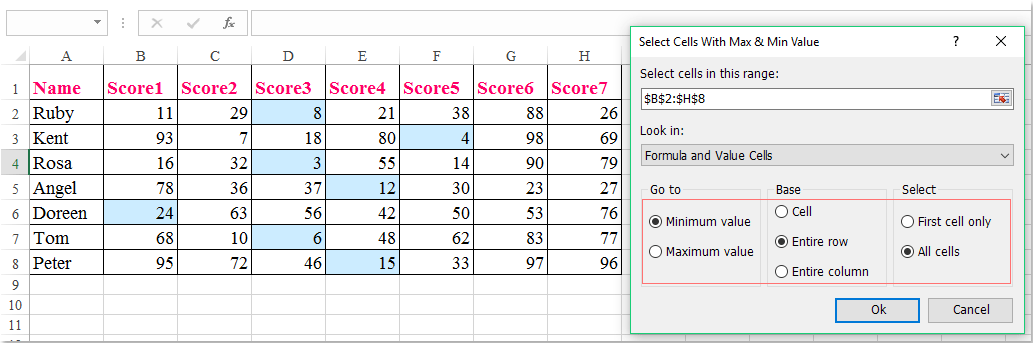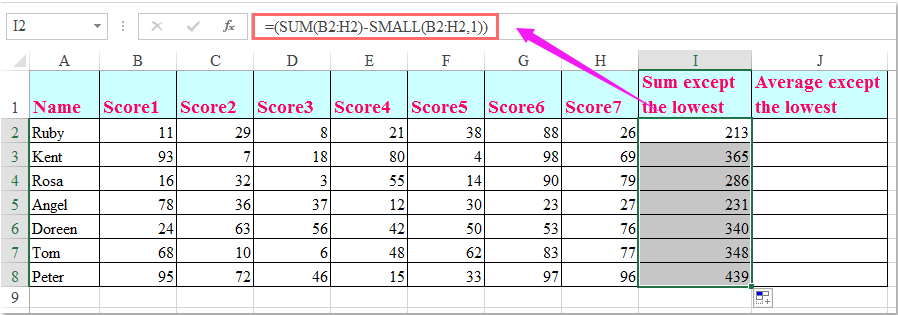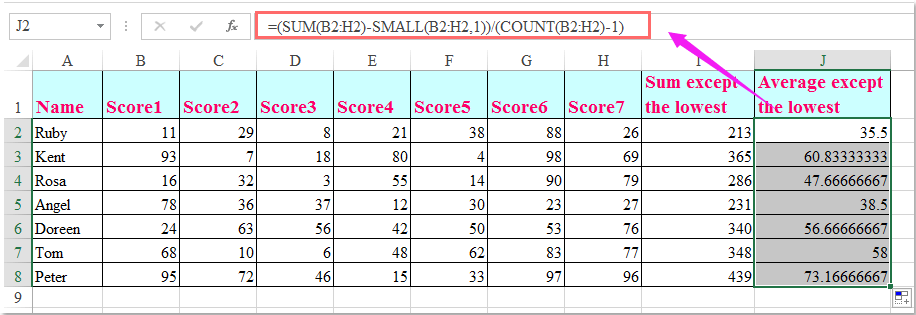Tip: Other languages are Google-Translated. You can visit the English version of this link.orRegisteror

## How to drop the lowest grade and get the average or total of values in Excel?

If you have a list of scores, now, you want to get the average and summation of the numbers but dropping the lowest or the lowest n numbers. This article, I will talk about some formulas to solve this task in Excel.

Drop the lowest grade and get the average or summation with formulas

 Select / highlight largest or smallest value in each column or row: Kutools for Excel's Select Cells with Max & Min Value utility can help you to select the largest or smallest cell value in a range of cells, in eaxh row or column as you need.Kutools for Excel: with more than 200 handy Excel add-ins, free to try with no limitation in 60 days.

####Drop the lowest grade and get the average or summation with formulas

Drop the lowest or lowest n numbers from the data list, and then average or sum the rest numbers, the following formulas can do you a favor, please do as this:

Sum the numbers but drop the lowest or lowest n numbers:

Please enter this formula: =(SUM(B2:H2)-SMALL(B2:H2,1)) into a blank cell where you want to output the result, and then drag the fill handle down to the cells that you want to apply this formula, and all the cells in each row have been added up ignoring the lowest number, see screenshot:Notes:

1. To sum the values but ignoring the lowest two, three or n numbers, please apply the following formulas:

=SUM(B2:H2)-SMALL(B2:H2,1)-SMALL(B2:H2,2)

=SUM(B2:H2)-SMALL(B2:H2,1)-SMALL(B2:H2,2)- SMALL(B2:H2,3)

=SUM(B2:H2)-SMALL(B2:H2,1)-SMALL(B2:H2,2)- SMALL(B2:H2,3)-……- SMALL(B2:H2,n)

2. In the above formulas, B2:H2 is the range that you want to sum, and the number 1, 2, 3…indicates which value is to be dropped from the list of numbers, 1 indicates that the smallest number should be dropped, 2 indicates that the second smallest number should be dropped, and so on.

Average the numbers but drop the lowest or lowest n numbers:

To get the average of the values but skip the lowest or lowest n numbers, the following formulas may do you a favor, please do as this:

Enter this formula: =(SUM(B2:H2)-SMALL(B2:H2,1))/(COUNT(B2:H2)-1) into a blank cell where you want to return the result, and drag the fill handle down to the cells that you want to apply this formula, and all the cells in each row have been averaged ignoring the lowest number, see screenshot:Notes:

1. To average the values but ignoring the lowest two, three or n numbers, please apply the following formulas:

=(SUM(B2:H2)-SMALL(B2:H2,1)-SMALL(B2:H2,2))/(COUNT(B2:H2)-2)

=(SUM(B2:H2)-SMALL(B2:H2,1)-SMALL(B2:H2,2)- SMALL(B2:H2,3))/(COUNT(B2:H2)-3)

=(SUM(B2:H2)-SMALL(B2:H2,1)-SMALL(B2:H2,2)- SMALL(B2:H2,3)-……- SMALL(B2:H2,n)) /(COUNT(B2:H2)-n)

2. In the above formulas, B2:H2 is the cells that you want to average, and the number 1, 2, 3…indicates which value is to be dropped from the list of numbers, 1 indicates that the smallest number should be dropped, 2 indicates that the second smallest number should be dropped, and so on.

### Kutools for Excel Solves Most of Your Problems, and Increases Your Productivity by 80%

• Reuse: Quickly insert complex formulas, charts and anything that you have used before; Encrypt Cells with password; Create Mailing List and send emails...
• Super Formula Bar (easily edit multiple lines of text and formula); Reading Layout (easily read and edit large numbers of cells); Paste to Filtered Range...
• Merge Cells/Rows/Columns without losing Data; Split Cells Content; Combine Duplicate Rows/Columns... Prevent Duplicate Cells; Compare Ranges...
• Select Duplicate or Unique Rows; Select Blank Rows (all cells are empty); Super Find and Fuzzy Find in Many Workbooks; Random Select...
• Exact Copy Multiple Cells without changing formula reference; Auto Create References to Multiple Sheets; Insert Bullets, Check Boxes and more...
• Extract Text, Add Text, Remove by Position, Remove Space; Create and Print Paging Subtotals; Convert Between Cells Content and Comments...
• Super Filter (save and apply filter schemes to other sheets); Advanced Sort by month/week/day, frequency and more; Special Filter by bold, italic...
• Combine Workbooks and WorkSheets; Merge Tables based on key columns; Split Data into Multiple Sheets; Batch Convert xls, xlsx and PDF...
• More than 300 powerful features. Supports Office/Excel 2007-2019 and 365. Supports all languages. Easy deploying in your enterprise or organization. Full features 30-day free trial.### Office Tab Brings Tabbed interface to Office, and Make Your Work Much Easier

• Enable tabbed editing and reading in Word, Excel, PowerPoint, Publisher, Access, Visio and Project.
• Open and create multiple documents in new tabs of the same window, rather than in new windows.
• Increases your productivity by 50%, and reduces hundreds of mouse clicks for you every day!Say something here...
symbols left.